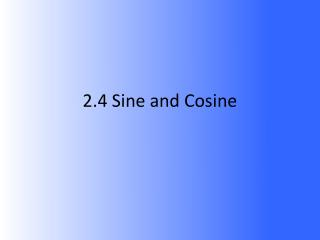DownloadDownload Presentation2.4 Sine and Cosine

# 2.4 Sine and Cosine

Télécharger la présentation## 2.4 Sine and Cosine

- - - - - - - - - - - - - - - - - - - - - - - - - - - E N D - - - - - - - - - - - - - - - - - - - - - - - - - - -
##### Presentation Transcript

1. 2.4 Sine and Cosine

2. Sine (Sin) sinX = opposite side hypotenuse 16 8 X

3. Cosine (Cos) cosX = adjacent side hypotenuse 16 X 13

4. S O H C A H T O A I N P P O S I T E Y P O T E N U S E O S D J A C E N T Y P O T E N U S E A N P P O S I T E D J A C E N T